36-402: Exam 1

Introduction

Traditionally, economists have categorized modern economic growth with the beginning of the Industrial Revolution in the early 1800’s. In this report, we will analyze what factors that led to economic growth in the pre-Industrial Revolution era, the primitive accumulation. We theorize that countries with access to Atlantic Trade had the greatest economic growth. We further theorize, that countries with relatively free institutions grew from greater access to Atlantic Trade and, therefore, greater economic growth in the primitive accumulation.

To test our theory, we will be using RAJ.csv, a data set containing economic and political information on countries during the primitive accumulation. There are 224 observations of the 14 variables: country, year, urbanization (fraction of population living in cities and towns) which will serve as our proxy for economic growth, population (thousands), ratio of country’s Atlantic coast-line (miles) to total land area (square miles), an increasing rating of constraint of the executive branch (1 - 7), a rating of prior constraint of the executive branch, index of the volume of Atlantic trade across all countries, indicators for countries in Western and Eastern Europe, the number of wars the country engaged in, indicator of a Protestant country, indicator of country belonging to the Holy Roman Empire and the estimate of per-capita GDP in current dollars. We have removed China, India, Japan and Turkey from the data set as they are missing essential data needed for the report and are non-European countries.

Initial Modeling

Our initial model, urb.lm, is a basic linear model with urbanization as the response and few of the other variables as predictors.

urb.lm <- lm(urbanization ~ factor(country) + factor(year) +
factor(westernEurope) + (atlTrade:coastToArea) + ordered(initialConstr) +

Table: Linear Model

The model’s coefficients are summarized below. Five of the countries have positive coefficients, meaning there would be greater urbanization than if not in that country; Albania, Belgium, Italy, Netherlands and Spain. As time passes, urbanization increases as expected. and the interaction between atlTrade and coastToArea is positive as expected. Interestingly, the coefficients of WesternEurope and initialConstr were NA, indicating a perfect colinearity with one of the other variables. Prior knowledge of the European theater tells us that any country with Atlantic Coastline would be a country in Western Europe as Eastern European countries are either not on the Atlantic Seaboard or are landlocked. We theorize that the collinearity for initialConstr might relate to the interaction term present. Further testing is needed, to investigate these effects.

Estimate Std. Error t value Pr(>|t|)
(Intercept) 0.1200 0.017 6.90 9.7e-11
factor(country)Austria -0.0670 0.020 -3.30 1.2e-03
factor(country)Belgium 0.1200 0.021 5.90 2.5e-08
factor(country)Bulgaria -0.0300 0.020 -1.50 1.4e-01
factor(country)Czech -0.0970 0.020 -4.80 3.9e-06
factor(country)Denmark -0.1200 0.034 -3.50 7.2e-04
factor(country)England -0.1100 0.033 -3.40 8.3e-04
factor(country)Finland -0.1100 0.020 -5.60 8.1e-08
factor(country)France -0.0430 0.021 -2.10 4.0e-02
factor(country)Germany -0.0440 0.026 -1.70 9.4e-02
factor(country)Greece -0.0650 0.020 -3.20 1.5e-03
factor(country)Hungary -0.0570 0.020 -2.90 5.0e-03
factor(country)Ireland -0.1300 0.023 -5.90 2.7e-08
factor(country)Italy 0.0670 0.020 3.30 1.0e-03
factor(country)Netherlands 0.0480 0.034 1.40 1.6e-01
factor(country)Norway -0.1000 0.030 -3.30 1.3e-03
factor(country)Poland -0.0930 0.020 -4.60 8.9e-06
factor(country)Portugal -0.0430 0.034 -1.30 2.0e-01
factor(country)Romania -0.0890 0.020 -4.40 1.8e-05
factor(country)Russia -0.0960 0.020 -4.70 4.7e-06
factor(country)Serbia -0.0850 0.020 -4.20 4.4e-05
factor(country)Spain 0.0280 0.021 1.30 1.9e-01
factor(country)Sweden -0.0870 0.020 -4.30 2.7e-05
factor(country)Switzerland -0.0650 0.020 -3.20 1.6e-03
factor(year)1400 0.0017 0.012 0.15 8.8e-01
factor(year)1500 0.0061 0.012 0.51 6.1e-01
factor(year)1600 0.0130 0.012 1.10 2.8e-01
factor(year)1700 0.0240 0.012 1.90 6.0e-02
factor(year)1750 0.0220 0.013 1.70 8.6e-02
factor(year)1800 0.0550 0.013 4.20 3.8e-05
factor(year)1850 0.0560 0.013 4.20 3.9e-05

Analysis of Initial Model

We will analyze how urb.lm fits the data, looking at outliers, influential points, and residuals before checking the cross-validated mean squared error.

Outliers

Below are the Rstudent and Cook’s Distance plots of our initial model. There are a few worrisome points, having Cook’s Distance greater than 0.05 and Rstudent above 2: Albania in 1800 where urbanization quadruples, Belgium post Black Plague (1400), where urbanization triples and England in 1850 where population doubles. A cursory glance of history does not reveal much about changes in Albania in 1800, however its Cook’s Distance is abnormally large and we will therefore remove it. There are ties between urbanization and the Black Plague. The Industrial Revolution begins in Britain in the 1800’s and thus should explains its outlier status. Those three points have been removed and the model rerun.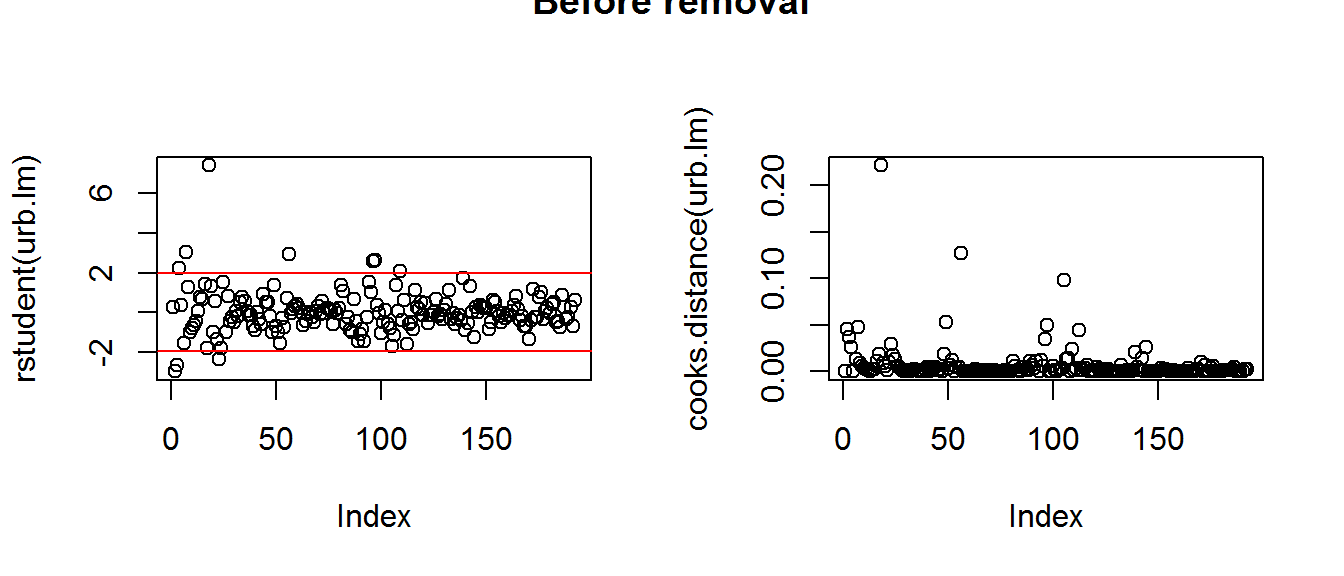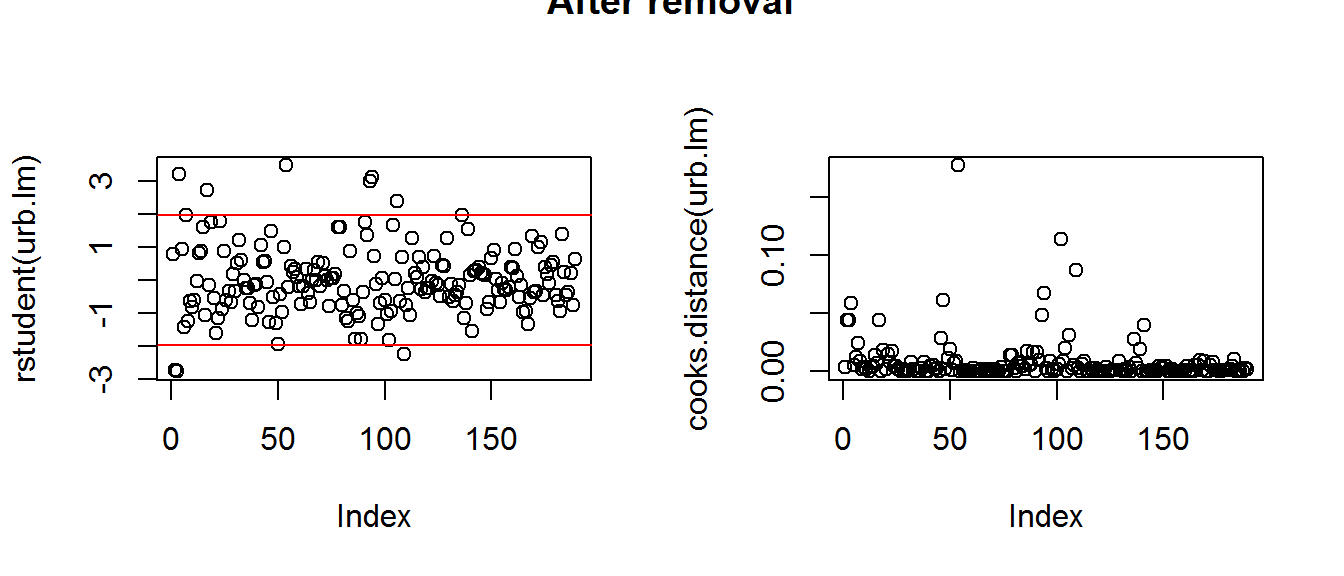Residuals

We have plotted the residuals and the Q-Q Plot of urb.lm. The residuals appeared without pattern but centered around 0.01. The Q-Q Plot appears semi-linear with discrepancies throughout. Neither of these plots are indicative of a strong model, further checking is required.

urb.lm.resid <- residuals(urb.lm)
urb.lm.fit <- fitted(urb.lm)
urb.lm.df <- data.frame(Residuals = urb.lm.resid, Fitted = urb.lm.fit)
#QQPlot of Residuals
urb.lm.sd <- sqrt(deviance(urb.lm)/df.residual(urb.lm))
urb.lm.residSD <- urb.lm$residuals/urb.lm.sd lm.res <- ggplot(urb.lm.df) + geom_point(aes(Fitted, Residuals)) + xlab('Fitted') + ylab('Residuals') + geom_hline(aes(yintercept = 0)) + ggtitle("Linear Model Residuals") #Plot the qqplot of the Sd's lm.qq <- ggplot() + geom_point(aes(qnorm(ppoints(urb.lm.residSD)), sort(urb.lm.residSD))) + xlab('Theoretical Quantiles') + ylab('Observed Quantiles') + geom_line(aes(qnorm(ppoints(urb.lm.residSD)), qnorm(ppoints(urb.lm.residSD)))) + ggtitle("Linear Model Q-Q Plot") grid.arrange(lm.res, lm.qq, nrow = 1)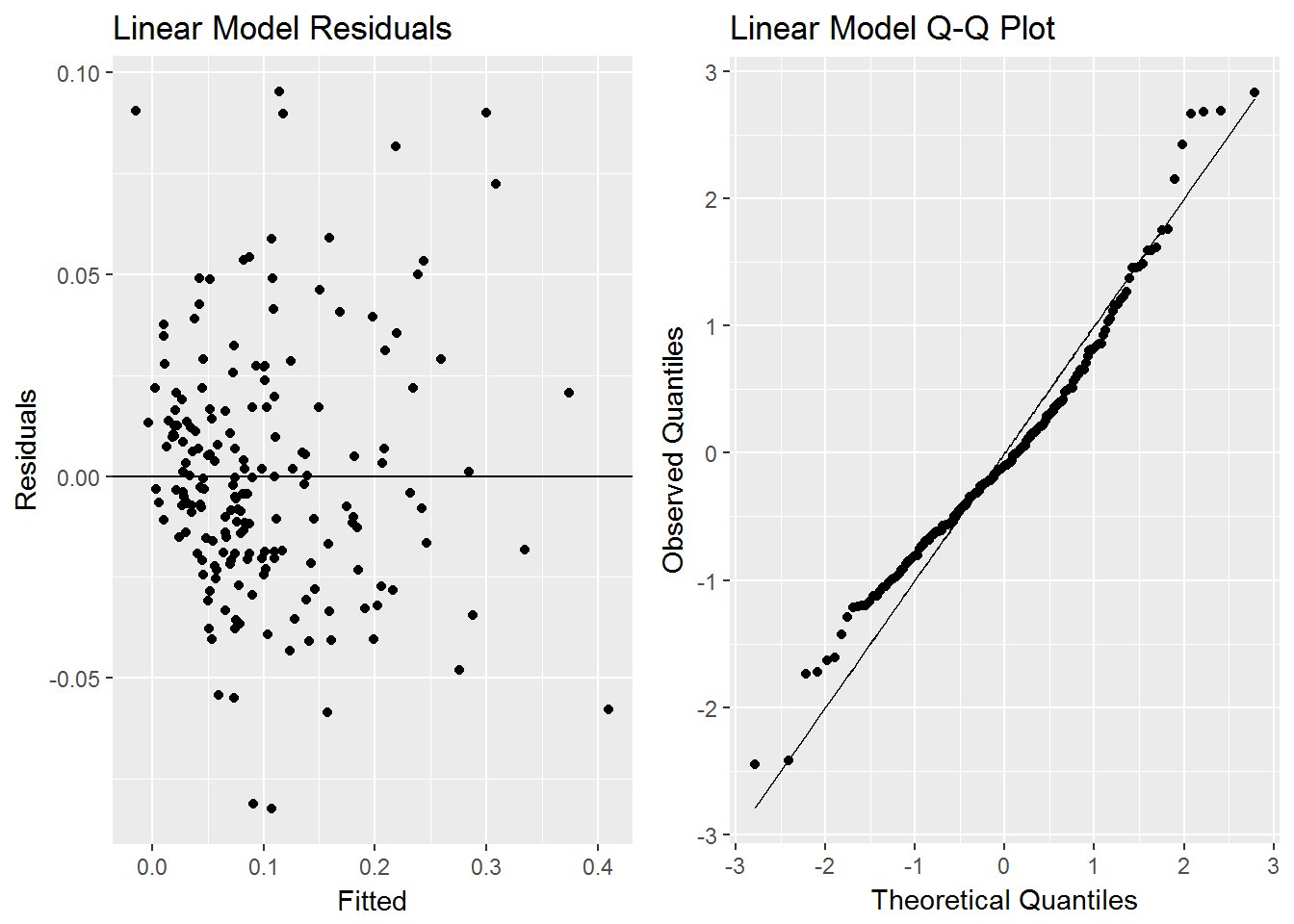Mean Squared Error #Professors Code cv.model <- function(data, model, formulae, nfolds=5) { data <- na.omit(data) formulae <- sapply(formulae, as.formula) n <- nrow(data) fold.labels <- sample(rep(1:nfolds, length=n)) mses <- matrix(NA, nrow=nfolds, ncol=length(formulae)) colnames <- as.character(formulae) for (fold in 1:nfolds) { test.rows <- which(fold.labels == fold) train <- data[-test.rows,] test <- data[test.rows,] for (form in 1:length(formulae)) { current.model <- model(formula=formulae[[form]], data=train) predictions <- predict(current.model, newdata=test) test.responses <- eval(formulae[[form]][], envir=test) test.errors <- test.responses - predictions mses[fold, form] <- mean(test.errors^2) } } return(colMeans(mses)) } mse.lm <- cv.model(raj, lm, "urbanization ~ factor(country) + factor(year) + factor(westernEurope) + (atlTrade:coastToArea) + ordered(initialConstr) + ordered(initialConstr):atlTrade:coastToArea") Instead of returnung the simple mean squared error, we felt it would be better suited to cross validate the mean squared error. Using 5-fold cross validation, our algorithm returns a mean squared error of 0.0015457. Secondary Modeling Introduction After testing a few different models, We chose to use a generalized linear model with different variables and interactions. Decision Trees To help decide which variables to use, we used a decision tree, to see which variables were important for urbanization. From our variable importance graph, we see that initalConstr is the most important variable followed by execConstr and year. Our least important variables were country, coastToArea and population. While the decision tree was useful for observing the importance of the variables, we did some brief testing of models without the least important variables, and they performed remarkably poorer than the final model we chose. #Decision Tree set.seed(100) urb.tree <- train(urbanization ~ ., data = raj, method = "ctree", trControl = trainControl(method = "cv", number = 5), tuneGrid = expand.grid(mincriterion = 0.95)) #Plotting important variables plot(varImp(urb.tree))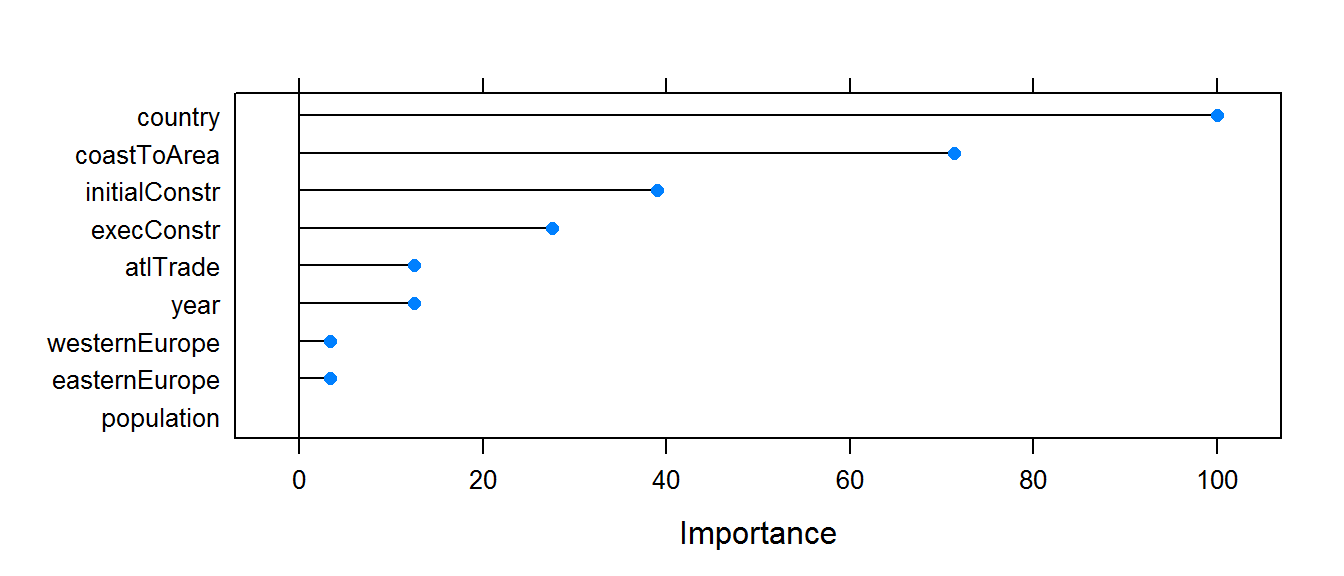Testing Models We tested a few different models cross-validated mean squared errors and chose the one with the smallest value. Below are the ones we tested. tm1 <- cv.model(raj, glm, "urbanization ~ factor(year) + (atlTrade:coastToArea) + ordered(initialConstr) + ordered(initialConstr):atlTrade:coastToArea") tm2 <- cv.model(raj, glm, "urbanization ~ factor(country) + (atlTrade:coastToArea) + ordered(initialConstr) + ordered(initialConstr):atlTrade:coastToArea") tm3 <- cv.model(raj, glm, "urbanization ~ factor(country) + (atlTrade:coastToArea) + ordered(initialConstr):atlTrade:coastToArea") tm4 <- cv.model(raj, glm, "urbanization ~ factor(country) + (atlTrade:coastToArea) + ordered(initialConstr):atlTrade") The first test model tm1, without country or westernEurope had a cross-validated mean squared error of 0.0029591. After tm1, we realized, while the decision tree did not see the importance of country, the model needed it to perform well. The removal of westernEurope had no effect on the model, probably due its collinearity. The second model, tm2, included country and removed year. Its cross-validated mean squared error was 0.0020516. While tm2 performed better than tm1, performed worse than urb.lm. Our third test model, tm3, removed initialConstr, its cross-validated mean square error was 0.0018385. While tm3 performed well, we had a hunch that removing the coastToArea interaction term could further improve the model. tm4 was our final test model, its cross-validated mean square error was 0.0017693 and was the best of all of the four models. We felt confident in tm4 to continue our analysis. Generalized Linear Model As mentioned above, we used tm4 as our final model. tm4, now referred to as urb.glm had some differences from urb.lm. We had removed the binary indicator for westernEurope due to its collinearity issues, as well as year as it did not have much of an effect on the model. We removed coastToArea from the larger interaction term, but left the interaction term between initialConstr and atlTrade as per our initial theory. We will continue to use the data with outliers removed based on our understanding that these points would still be outliers and overly influential. The model is written below. urb.glm <- glm(urbanization ~ factor(country) + (atlTrade:coastToArea) + atlTrade:ordered(initialConstr), data = raj) Table: GLM urb.glm’s coefficients are summarized below. Similarly to urb.lm, only five countries have positive coefficients. There is one insignificant country; Bulgaria. The interaction between atlTrade and areaToCoast is still significant and has a positive coefficient, indicating greater urbanization (economic growth) per their interaction. Our new variable, the interaction between atlTrade and initialConstr is significant on all levels with a negative coefficient on the low level, but positive effects on the other levels. Interestingly, it appears that there is a point of initial executive constraint at which its influence no longer changes and the coefficient stabilizes between levels. Estimate Std. Error t value Pr(>|t|) (Intercept) 0.0960 0.0150 6.20 3.8e-09 factor(country)Austria -0.0480 0.0190 -2.60 1.1e-02 factor(country)Belgium 0.0980 0.0240 4.10 7.9e-05 factor(country)Bulgaria -0.0110 0.0190 -0.59 5.6e-01 factor(country)Czech -0.0790 0.0230 -3.50 5.6e-04 factor(country)Denmark -0.1600 0.0240 -6.70 3.6e-10 factor(country)England -0.1100 0.0280 -3.80 1.7e-04 factor(country)Finland -0.0950 0.0190 -4.90 2.1e-06 factor(country)France -0.0370 0.0230 -1.60 1.0e-01 factor(country)Germany -0.0400 0.0190 -2.10 3.4e-02 factor(country)Greece -0.0470 0.0190 -2.50 1.3e-02 factor(country)Hungary -0.0400 0.0230 -1.80 7.6e-02 factor(country)Ireland -0.0530 0.0250 -2.10 3.6e-02 factor(country)Italy 0.0720 0.0230 3.10 2.2e-03 factor(country)Netherlands 0.0880 0.0240 3.70 3.5e-04 factor(country)Norway -0.1000 0.0190 -5.40 2.5e-07 factor(country)Poland -0.0880 0.0230 -3.80 2.1e-04 factor(country)Portugal -0.0690 0.0250 -2.70 7.1e-03 factor(country)Romania -0.0710 0.0190 -3.80 2.3e-04 factor(country)Russia -0.0770 0.0190 -4.10 6.0e-05 factor(country)Serbia -0.0660 0.0190 -3.50 5.2e-04 factor(country)Spain 0.0480 0.0240 2.00 4.7e-02 factor(country)Sweden -0.0510 0.0240 -2.20 3.3e-02 factor(country)Switzerland -0.0480 0.0230 -2.10 3.6e-02 atlTrade:coastToArea 1.2000 0.0920 13.00 1.0e-25 atlTrade:ordered(initialConstr).L -0.0050 0.0025 -2.00 4.8e-02 atlTrade:ordered(initialConstr).Q 0.0094 0.0025 3.70 2.6e-04 atlTrade:ordered(initialConstr).C 0.0150 0.0035 4.20 3.8e-05 atlTrade:ordered(initialConstr)^4 0.0150 0.0029 5.20 7.2e-07 Analysis of Secondary Model Introduction Like we did with urb.lm, we analyzed urb.glm, Looking at outliers, influential points, residuals before checking the cross-validated mean squared error. After checking urb.glm, we compared the two models. Outliers Below are the rstudent and Cooks Distance plots of urb.glm. Since we have removed the most worrisome outliers with urb.lm, the rstudent and Cook’s Distance plot do not show many worrisome points. #Review Rstudent par(mfrow = c(1,2)) plot(rstudent(urb.glm)) abline(h=qt(.025,df=nrow(raj)-2),col="red") abline(h=qt(.975,df=nrow(raj)-2),col="red") #cook's distance plot(cooks.distance(urb.glm)) title("After Removal", outer = TRUE)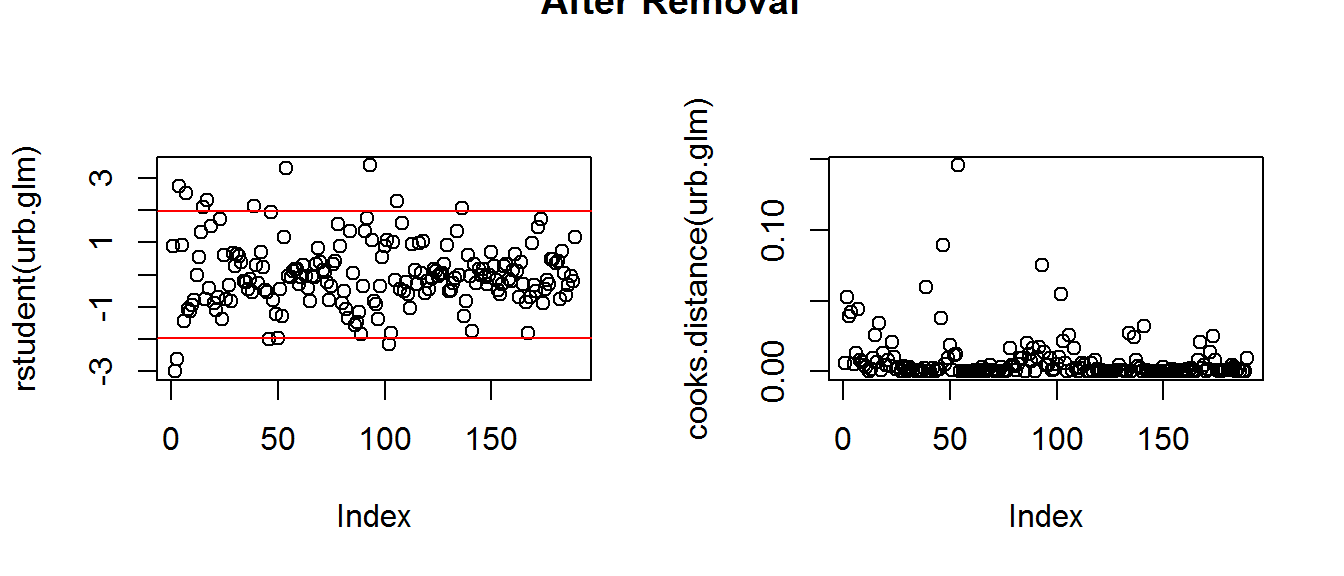Residuals Below we have plotted the residuals and a Q-Q plot of urb.glm. Our residuals appear patternless and centered around zero. Our Q-Qplot appears mostly linear with some variability on the right tail. urb.glm.resid <- residuals(urb.glm) urb.glm.fit <- fitted(urb.glm) urb.glm.df <- data.frame(Residuals = urb.glm.resid, Fitted = urb.glm.fit) #QQPlot of Residuals urb.glm.sd <- sqrt(deviance(urb.glm)/df.residual(urb.glm)) urb.glm.residSD <- urb.glm$residuals/urb.glm.sd

glm.res <- ggplot(urb.glm.df) +
geom_point(aes(Fitted, Residuals)) +
xlab('Fitted') +
ylab('Residuals') +
geom_hline(aes(yintercept = 0)) +
ggtitle("Linear Model Residuals")

#Plot the qqplot of the Sd's
glm.qq <- ggplot() + geom_point(aes(qnorm(ppoints(urb.glm.residSD)),
sort(urb.glm.residSD))) +
xlab('Theoretical Quantiles') +
ylab('Observed Quantiles') +
geom_line(aes(qnorm(ppoints(urb.glm.residSD)),
qnorm(ppoints(urb.glm.residSD)))) +
ggtitle("Linear Model Q-Q Plot")
grid.arrange(glm.res, glm.qq, nrow = 1)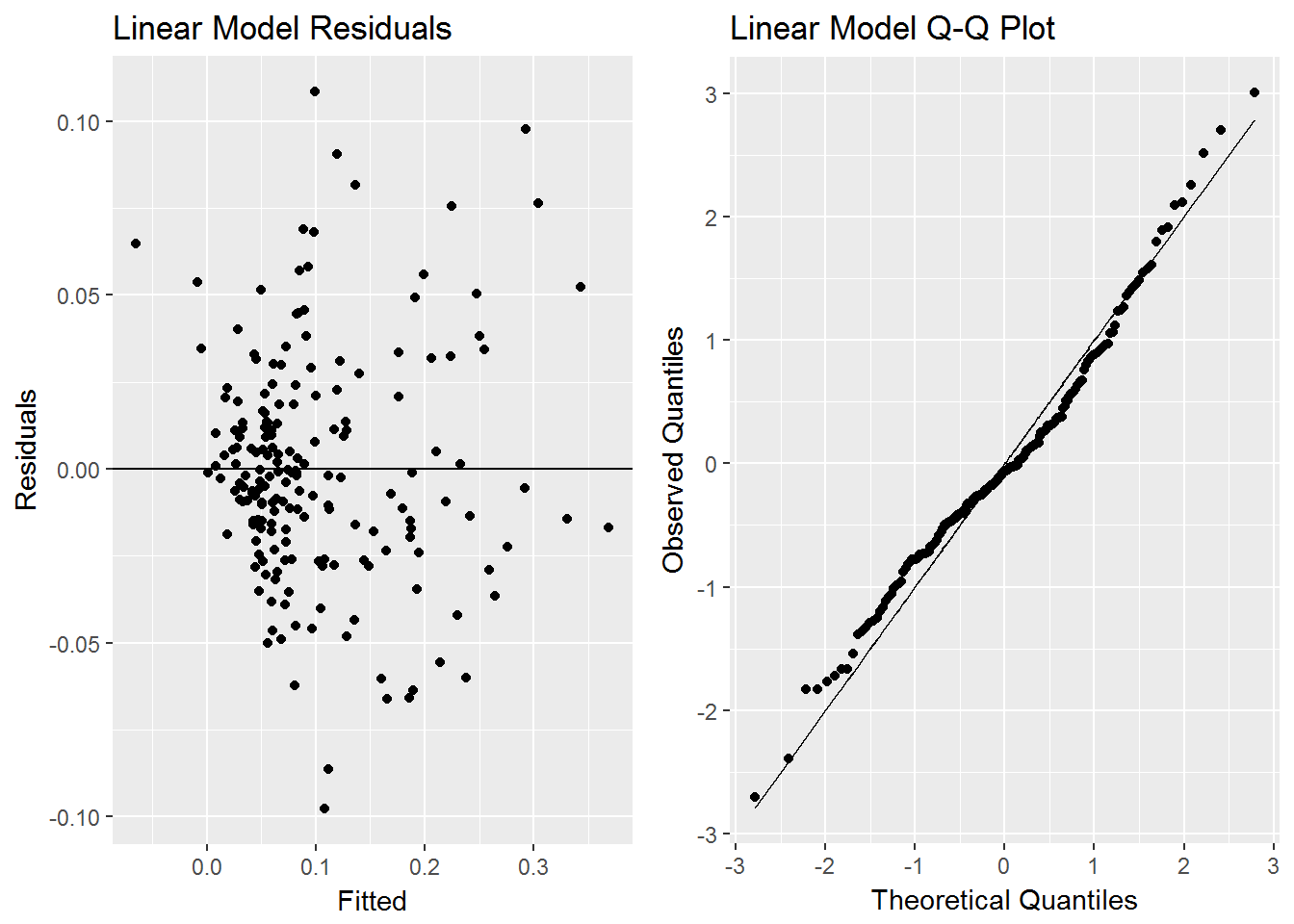Mean Squared Error

Using the function we created earlier, urb.glm has a cross-validated mean squared error of 0.0017667.

Comparing Models

Introduction

After analyzing urb.lm and urb.glm separately, we decided to compare the two models with a few different techniques.

resample <- function(x){
return(sample(x, size = length(x), replace = TRUE))
}

resampler.res <- function(model, column) {
temp.frame <- raj
new.data <- fitted(model) + resample(residuals(model))
temp.frame[,column] <- new.data
return(temp.frame)
}

joint.estimator <- function(dat){
fit.lm <- lm(dat[,"urbanization"] ~ factor(dat[,"country"]) + factor(dat[,"year"]) +
factor(dat[,"westernEurope"]) + ordered(dat[,"initialConstr"]) +
mse.lm <- mean(fit.lm$residuals^2) fit.glm <- glm(dat[,"urbanization"] ~ factor(dat[,"country"]) + (dat[,"atlTrade"]:dat[,"coastToArea"]) + dat[,"atlTrade"]:ordered(dat[,"initialConstr"])) mse.lm <- mean(fit.glm$residuals^2)
return(mse.lm - mse.glm)
}

#bootstrap for CI's
mse.bootstrap <- function(B, model1, model2, column) {
t.hat = mean(model1$residuals^2) - mean(model2$residuals^2)

boots <- replicate(B, joint.estimator(resampler.res(model1, column)))

return((sum(boots >= t.hat) + 1)/ (B + 1))
}

pv <- mse.bootstrap(1000, urb.lm, urb.glm, "urbanization")

Testing Models

We initially tested the differences between the two models with the use of Akaike Information Criterion and Bayesian Information Criterion, in both tests urb.glm returned a better result than urb.lm. We compared the two Q-Q plots side by side, and urb.glm’s plot appears more linear, while urb.lm’s has severe discrepancies near the tails and the entirety of the right-hand side.

Further Testing

While our preliminary testing had given us evidence that urb.glm was the better model, we decided additional testing was necessary. We ran a hypothesis test between bootstrapped mean squared errors. The probability under the basic linear model of observing a large gap in mean squared errors is 0.0009. Using an alpha level of 0.05, we find the result significant. Meaning, there is evidence to suggest that urb.lm does worse than urb.glm.

Conclusion

We have tested and compared our two models. While urb.lm performed well according to our cross-validated mean squared error, it has severe issues with collinearity. urb.glm, Performed better on all of our testing, residuals and mean squared error. All results give credence, to our belief that urb.glm is a better model than urb.lm.

Understanding the model

Our initial theory is well explained by urb.glm. Our original theory posited that economic growth pre-Industrial Revolution is best explained by Atlantic trade and a free society. urb.glm Included significant terms for Atlantic Trade both in interaction with Atlantic coastal area and with initial executive constraint in a country. Similarly, the initial constraint in interaction with Atlantic trade is significant at all levels, indicating its importance to the model and evidence to our theory. Interestingly the coefficient for initial constraint begins negative, moves positive and levels out. We believe that this indicates that there is a limit to how free a country can be at which point executive constraint does not affect economic growth. Conversely, having little executive constraint negatively affects economic growth. We have found evidence to suggest that economic growth during the primitive accumulation was driven by Atlantic trade and executive constraint.